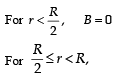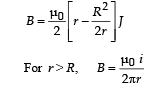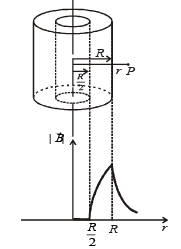Courses

# Single Correct MCQ Of Moving Charges And Magnetism, Past Year Questions JEE Advance, Class 12, Physics

## 24 Questions MCQ Test Class 12 Physics 35 Years JEE Mains &Advance Past year Paper | Single Correct MCQ Of Moving Charges And Magnetism, Past Year Questions JEE Advance, Class 12, Physics

Description
This mock test of Single Correct MCQ Of Moving Charges And Magnetism, Past Year Questions JEE Advance, Class 12, Physics for JEE helps you for every JEE entrance exam. This contains 24 Multiple Choice Questions for JEE Single Correct MCQ Of Moving Charges And Magnetism, Past Year Questions JEE Advance, Class 12, Physics (mcq) to study with solutions a complete question bank. The solved questions answers in this Single Correct MCQ Of Moving Charges And Magnetism, Past Year Questions JEE Advance, Class 12, Physics quiz give you a good mix of easy questions and tough questions. JEE students definitely take this Single Correct MCQ Of Moving Charges And Magnetism, Past Year Questions JEE Advance, Class 12, Physics exercise for a better result in the exam. You can find other Single Correct MCQ Of Moving Charges And Magnetism, Past Year Questions JEE Advance, Class 12, Physics extra questions, long questions & short questions for JEE on EduRev as well by searching above.
QUESTION: 1

### A conducting circular loop of radius r carries a constant current i. It is placed in a uniform magnetic field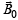such thatis perpendicular to the plane of the loop. The magnetic force acting on the loop is

Solution:

The magnetic field is perpendicular to the plane of the paper. Let us consider two diametrically opposite elements. By Fleming's left hand rule, on element AB the direction of force will be leftwards and the magnitude will be

dF = I ( d l)Bsin 90°= I ( dl)B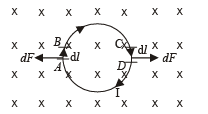On element CD, the direction of force will be towards right on the plane of the paper and the magnitude will be

dF = I ( d l)B.
These two forces will cancel out.
NOTE : Similarly, all forces acting on the diametrically opposite elements will cancel out in pair. The net force acting on the loop will be zero.

QUESTION: 2

### A battery is connected between two points A and B on the circumference of a uniform conducting ring of radius r and resistance R. One of the arcs AB of the ring subtends an angle q at the centre.The value of the magnetic induction at the centre due to the current in the ring is

Solution: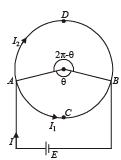Magnetic field at the centre due to current in arc ABC is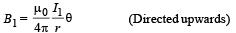Magnetic field at the centre due to current in arc ADB is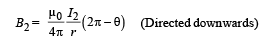Therefore net magnetic field at the centre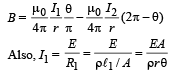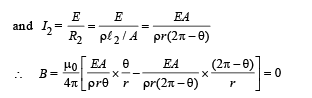QUESTION: 3

### A proton, a deuteron and an α - particle having the same kinetic energy are moving in circular trajectories in a constant magnetic field. If rp, rd, and ra denote respectively the radii of the trajectories of these particles, then

Solution:

KEY CONCEPT :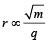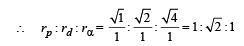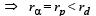QUESTION: 4

A circular loop of radius R, carrying current I, lies in x-y plane with its centre at origin. The total magnetic flux through x-y plane is

Solution:

The magnetic lines of force created due to current will be in such a way that on x – y plane these lines will be perpendicular. Further, these lines will be in circular loops. The number of lines moving downwards in x – y plane will be same in number to that coming upwards of the x – y plane. Therefore, the net flux will be zero.
One such magnetic line is shown in the figure.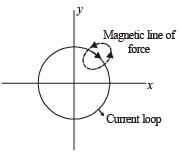QUESTION: 5

A charged particle is released from rest in a region of steady and uniform electric and magnetic fields which are parallel to each other. The particle will move in a

Solution: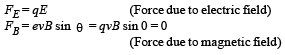Force due to electric field will make the charged particle released from rest to move in the straight line (that of electric field). Since the force due to magnetic field is zero, therefore, the charged particle will move in a straight line.

QUESTION: 6

A particle of charge q and mass m moves in a circular orbit of radius r with angular speed ω . The ratio of the magnitude of its magnetic moment to that of its angular momentum depen ds on

Solution:

The angular momentum L of the particle is given by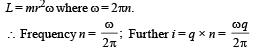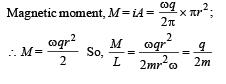QUESTION: 7

Two long parallel wires are at a distance 2d apart. They carry steady equal currents flowing out of the plane of the paper, as shown. The variation of the magnetic field B along the line XX' is given by

Solution:

The wires at A and B are perpendicular to the plane of paper and current is towards the reader. Let us consider certain points.
Point C (mid point between A and B) : The magnetic field at C due to A (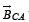) is in upward direction but magnetic field at C due to B is in downward direction.
Net field is zero.
Point E : Magnetic field due to A is upward and magnetic field due to B is downward but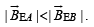∴Net magnetic field is in downward direction.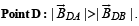Net field upwards. Similarly,, other points can be considered.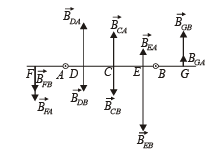QUESTION: 8

An infinitely long conductor PQR is bent to form a right angle as shown in Figure. A current I flows through PQR. The magnetic field due to this current at the point M is H1. Now, another infinitely long straight conductor QS is connected at Q so that current is I/2 in QR as well as in QS, the current in PQ remaining unchanged. The magnetic field at M is now H2. The ratio H1/H2 is given by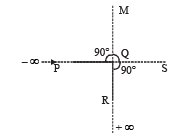Solution:

Case 1 : Magnetic field at M due to PQ and QR is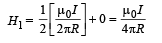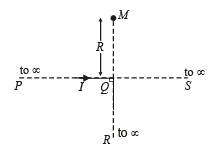Case 2 : When wire QS is joined.
H2 = (Magnetic field at M due to PQ) + (magnetic field at M due to QR) + (Magnetic field at M due to QS)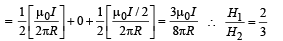NOTE : The magnetic field due to an infinitely long wire carrying current at a distance R from the end point is half that at a distance R from the middle point.

QUESTION: 9

An ionized gas contains both positive and negative ions. If it is subjected simultaneously to an electric field along the +x-direction and a magnetic field along the +z-direction, then

Solution:

Case of positively charged particle : Two forces are acting on the positively charged particle (a) due to electric field in the positive x-direction. (b) Force due to magnetic field.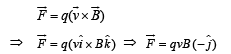This forces will move the positively charged particle towards Y-axis.

Case of negatively charged particle.
Two forces are acting on the negatively charged particle (a) due to electric field in the negative X-direction. (b) due to magnetic field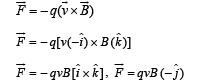Same direction as that of positive charge. (c) is the correct answer.

QUESTION: 10

A non-planar loop of conducting wire carrying a current I is placed as shown in the figure. Each of the straight sections of the loop is of length 2a. The magnetic field due to this loop at the point P(a, 0, a) points in the direction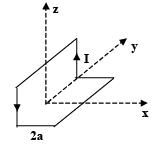Solution:

NOTE : If we take individual length for the purpose of calculating the magnetic field in a 3-Dimensional figure then it will be difficult.
Here a smart choice is divide the loop into two loops.
One loop is ADEFA in y-z plane and the other loop will be ABCDA in the x – y plane.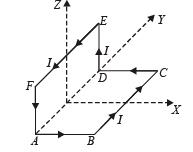We actually do not have any current in the segment AD. By choosing the loops we find that in one loop we have to take current from A to D and in the other one from D to A. Hence these two cancel out the effect of each other as far as creating magnetic field at the concerned point P is considered.
The point (a, 0, a) is in the X-Z plane.
The magnetic field due to current in ABCDA will be in + ve Z-direction.

NOTE : Due to symmetry the y-components and xcomponents will cancel out each other.
Similarly the magnetic field due to current in ADEFA will be  in x-direction.

∴ The resultant magnetic field will be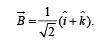QUESTION: 11

Two particles A and B of masses mA and mB respectively and having the same charge are moving in a plane. A uniform magnetic field exists perpendicular to this plane. The speeds of the particles are vA and vB respectively and the trajectories are as shown in the figure. Then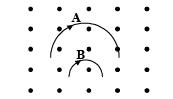Solution:

KEY CONCEPT : When a charged particle is moving at right angles to the magnetic field then a force acts on it which behaves as a centripetal force and moves the particle in circular motion.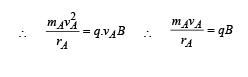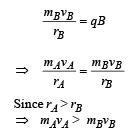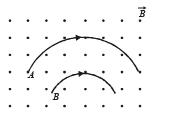QUESTION: 12

A coil having N turns is wound tightly in the form of a spiral with inner and outer radii a and b respectively. When a current I passes through the coil, the magnetic field at the center is

Solution:

Let us consider a thickness dx of wire. Let it be at a distance x from the centre O.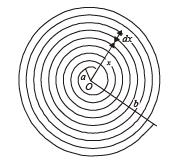Number of turns per unit length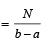∴ Number of turns in thickness dx =dx

Small amount of magnetic field is produced at O due to thickness dx of the wire.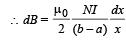On integrating, we get,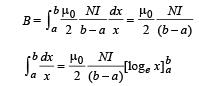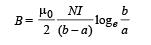QUESTION: 13

A particle of mass m and charge q moves with a constant velocity v along the positive x-direction. It enters a region containing a uniform magnetic field B directed along the negative z-direction, extending from x = a to x = b. The minimum value of v required so that the particle can just enter the region x > b is

Solution: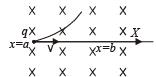Width of the magnetic field region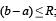where 'R' is its radius of curvature inside magnetic field,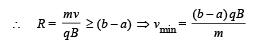QUESTION: 14

A long straight wire along the Z-axis carries a current I in the negative Z-direction. The magnetic vector field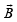at a point having coordinates (x, y) in the Z = 0 plane is

Solution:

The wire carries a current I in the negative z-direction.
We have to consider the magnetic vector field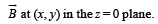Magnetic fieldis perpendicular to OP.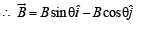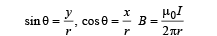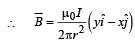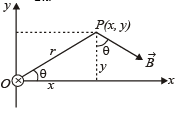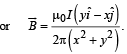QUESTION: 15

The magnetic field lines due to a bar magnet are correctly shown in

Solution:

NOTE : Magnetic lines of force form closed loops.
Inside a magnet, these are directed from south to north pole.

QUESTION: 16

For a positively charged particle moving in a x-y plane initially along the x-axis, there is a sudden change in its path due to the presence of electric and/or magnetic fields beyond P.
The curved path is shown in the x-y plane and is found to be non-circular. Which one of the following combinations is possible?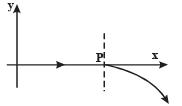Solution:

The velocity at P is in the X-direction (given).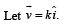After P, the positively charged particle gets deflected in the x – y plane toward – y direction and the path is non-circular.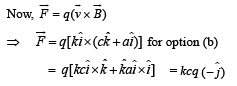Since in option (b), electric field is also present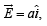therefore it will also exert a force in the + X direction.
The net result of the two forces will be a non-circular path.
Only option (b) fits for the above logic. For other option, we get some other results.

QUESTION: 17

A conducting loop carrying a current I is placed in a uniform magnetic field pointing into the plane of the paper as shown. The loop will have a tendency to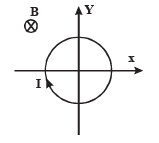Solution:

KEY CONCEPT : Use Fleming's left hand rule. We find that a force is acting in the radially outward direction throughout the circumference of the conducting loop.

QUESTION: 18

A  current carrying loop is placed in a uniform magnetic field in four different orientations, I, II, III & IV arrange them in the decreasing order of Potential Energy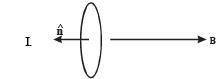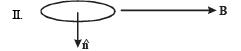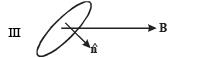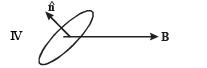Solution: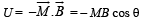In case I, θ = 180°, U = + MB

In case II, θ = 90°, U = 0

In case III, θ= acute, U = + ve (less than  + MB)

In case IV, θ= obtuse, U = – ve

∴ I > III > II > IV.

QUESTION: 19

An electron travelling with a speed u along th e positive xaxis enters into a region of magnetic field where B = –B0 kˆ (x > 0). It comes out of the  region with speed v then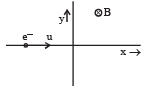Solution:

The force acting on electron will be perpendicular to the direction of velocity till the electron remains in the magnetic field. So the electron will follow the path as given.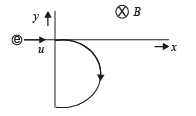QUESTION: 20

A magnetic field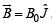exists in the region a < x < 2a, and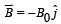, in the region 2a < x < 3a, where B0 is a positive constant. A positive point charge moving with a velocity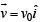, where v0 is a positive constant, enters the magnetic field at x = a. The trajectory of the charge in this region can be like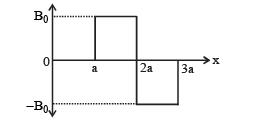Solution:

Use the vector for m of B and v in the formulae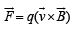to get the instantaneous direction of force at x = a and x = 2a.

QUESTION: 21

A thin flexible wire of length L is connected to two adjacent fixed points and carries a current I in the clockwise direction, as shown in the figure. When the system is put in a uniform magnetic field of strength B going into the plane of the paper, the wire takes the shape of a circle. The tension in the wire is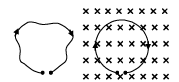Solution:

Let us consider an elemental length dl subtending an angle dθ at the centre of the circle. Let FB be the magnetic force acting on this length. Then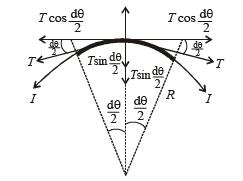FB = BI (dl)  directed upwards as shown

= BI (Rdθ)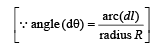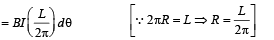Let T be the tension in the wire acting along both ends of the elemental length as shown. On resolving T, we find that the components.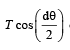cancel out and
the components.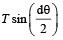add up to balance FB.
At equilibrium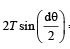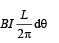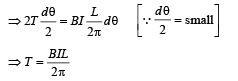QUESTION: 22

A long insulated copper wire is closely wound as a spiral of ‘N’ turns. The spiral has inner radius ‘a’ and outer radius ‘b’. The spiral lies in the XY plane and a steady current ‘I’ flows through the wire. The Z-component of the magnetic field at the centre of the spiral is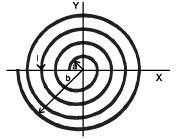Solution:
QUESTION: 23

A loop carrying current I lies in the x-y plane as shown in the figure. The unit vector kˆ is coming out of the plane of the paper. The magnetic moment of the current loop is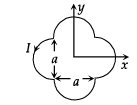Solution:

The magnetic moment of a current carrying loop is given by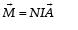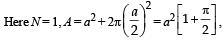the direction is towards positive z-axis.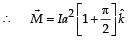QUESTION: 24

An infinitely long hollow conducting cylinder with inner radius R/2 and outer radius R carries a uniform current density along its length. The magnitude of the magnetic field,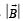as a function of the radial distance r from the axis is best represented by

Solution: